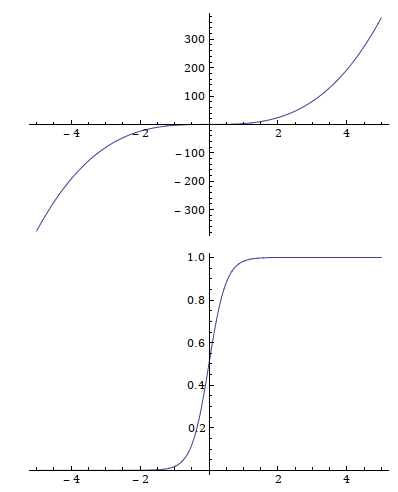## Floating point representation and error analysis

Floating point representation and error analysis

A real number can have infinite number of decimal digits while we only have a finite number of bits on a computer, this makes things look a little weird at times, for example:

The practical way of representing a real number is to represent the significant digits and the exponent:

Note that this makes our representation unevenly spaced, i.e. they are more dense near zero, imagine:

\begin{align*} 1.1 &- 1.0 \\ 1.1e3 &- 1.0e3 \end{align*}

The part we fail to represent is error. Sources of error include:

Example of discretization: numberical differentiation. We want $\lim_{d\rightarrow 0} (f(x+d)-f(x))/d$, but we cannot get d to be infinitely small in a machine, so we can only get a small enough d and have to settle with it.

There are way to contain errors, for example:

Errors are difficult to measure directly, because the true value is unknown to the machine in the first place. But it can be measured in indirect ways, for instance:

For example, in root finding, we need $x$ satisfying $f(x) = 0$, we can get $f(x_{est}) \approx 0$. To measure $x - x_{est}$, we indirectly measure $f(x) - f(x_{est})$.

Another example of backward error: linear systems. We want to solve $Ax = b$ for $x$, we get $Ax_1 \approx b$, instead of (the unknown) $x - x_1$, we measure $(|Ax_1 - Ax|)^2$. (This is sometimes called Squared Sum of Residuals in statistics.) Forward error is the direct measure.

Condition number is defined this way:

\begin{align*} \text{condition} &= \frac{\text{forward error}}{\text{backward error}} \end{align*}In the above figures, the first curve has a large condition number and is said to be ill-conditioned, the second is said to be well-conditioned.